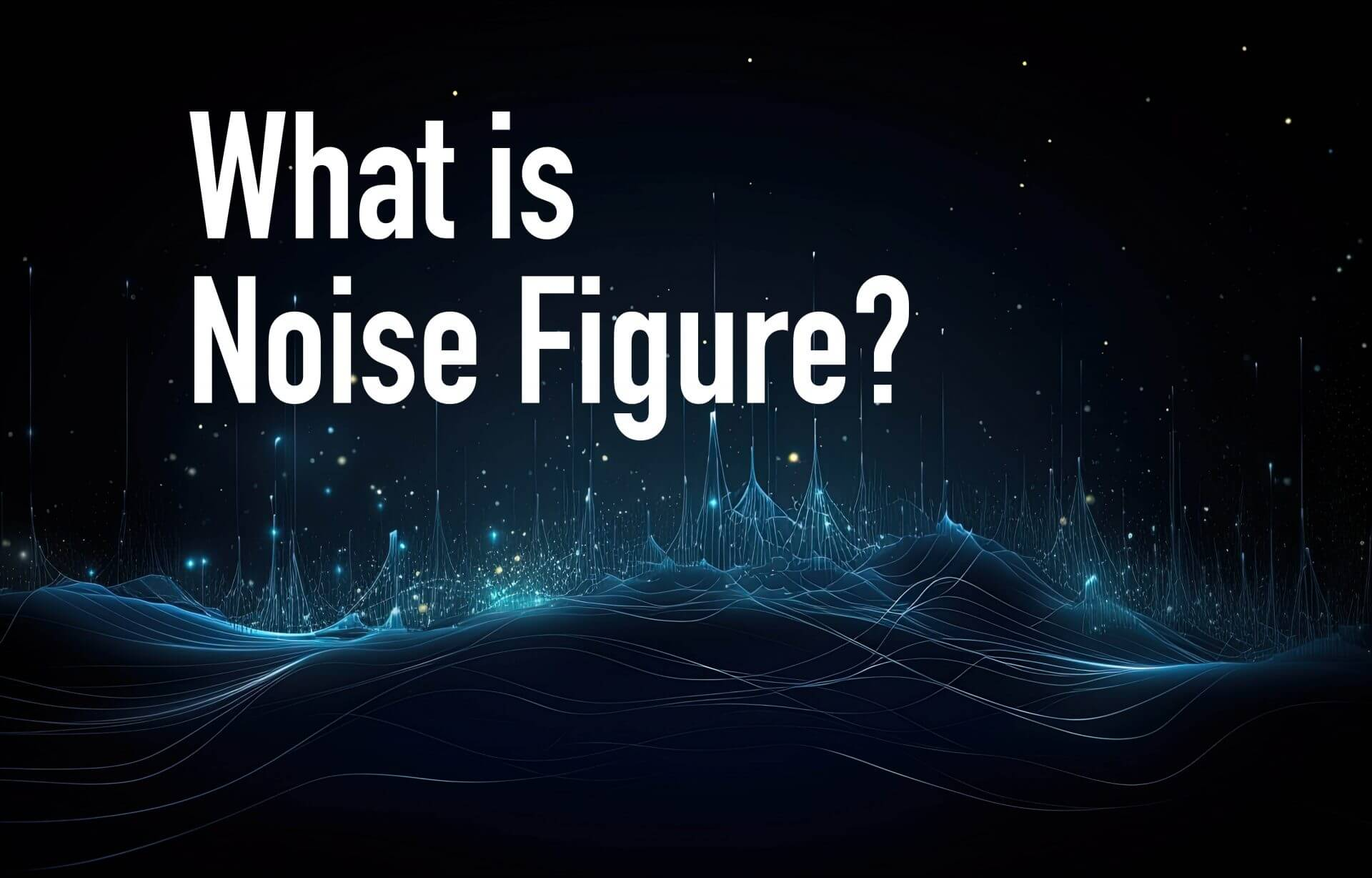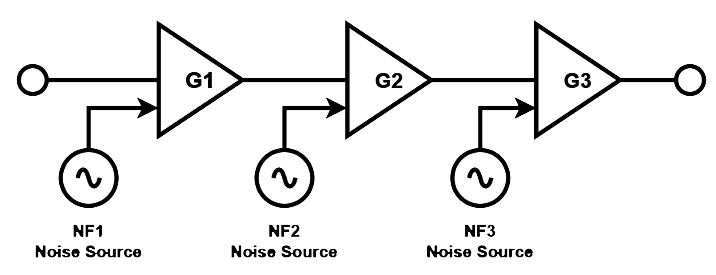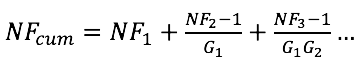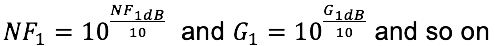|
|### What is Noise Figure?

March 22, 2023

Amplifiers suffer the effects of thermal noise and thermal charge movement within an amplifier appears on the output as added noise. This noise may be modeled as an external noise input to the amplifier. For example, the noise on the output of a perfect 10 dB amplifier in a 1 Hz bandwidth would be -164 dBm with no other signal input. If the actual noise output is -162 dBm, then the noise figure of the amplifier is said to be 2 dB.  A chain of amplifiers will exhibit cumulative noise.Figure 1 – Amplifier Chain with Noise

Cumulative noise figure is calculated as follows:Where the gains and noise figures are evaluated in linear terms. That is:A chain of three amplifiers, all with 10 dB of gain and 3 dB noise figure, would have a cumulative noise figure of 3.2 dB. It is clear from the formula that, as long as the gain of the first amplifier is relatively high, its noise figure will dominate the cumulative noise of the chain. A significant amount of attenuation introduced after the first amplifier would reduce its effective gain and allow the noise figure of the second amplifier to have a larger effect.

See this and more important radio frequency charts and formulas in this comprehensive application note